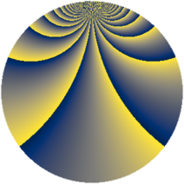# Properties

 Label 512.2.oLevel $512$ Weight $2$ Character orbit 512.o Rep. character $\chi_{512}(5,\cdot)$ Character field $\Q(\zeta_{128})$ Dimension $4032$ Newform subspaces $1$ Sturm bound $128$ Trace bound $0$

# Related objects

## Defining parameters

 Level: $$N$$ $$=$$ $$512 = 2^{9}$$ Weight: $$k$$ $$=$$ $$2$$ Character orbit: $$[\chi]$$ $$=$$ 512.o (of order $$128$$ and degree $$64$$) Character conductor: $$\operatorname{cond}(\chi)$$ $$=$$ $$512$$ Character field: $$\Q(\zeta_{128})$$ Newform subspaces: $$1$$ Sturm bound: $$128$$ Trace bound: $$0$$

## Dimensions

The following table gives the dimensions of various subspaces of $$M_{2}(512, [\chi])$$.

Total New Old
Modular forms 4160 4160 0
Cusp forms 4032 4032 0
Eisenstein series 128 128 0

## Trace form

 $$4032q - 64q^{2} - 64q^{3} - 64q^{4} - 64q^{5} - 64q^{6} - 64q^{7} - 64q^{8} - 64q^{9} + O(q^{10})$$ $$4032q - 64q^{2} - 64q^{3} - 64q^{4} - 64q^{5} - 64q^{6} - 64q^{7} - 64q^{8} - 64q^{9} - 64q^{10} - 64q^{11} - 64q^{12} - 64q^{13} - 64q^{14} - 64q^{15} - 64q^{16} - 64q^{17} - 64q^{18} - 64q^{19} - 64q^{20} - 64q^{21} - 64q^{22} - 64q^{23} - 64q^{24} - 64q^{25} - 64q^{26} - 64q^{27} - 64q^{28} - 64q^{29} - 64q^{30} - 64q^{31} - 64q^{32} - 64q^{33} - 64q^{34} - 64q^{35} - 64q^{36} - 64q^{37} - 64q^{38} - 64q^{39} - 64q^{40} - 64q^{41} - 64q^{42} - 64q^{43} - 64q^{44} - 64q^{45} - 64q^{46} - 64q^{47} - 64q^{48} - 64q^{49} - 64q^{50} - 64q^{51} - 64q^{52} - 64q^{53} - 64q^{54} - 64q^{55} - 64q^{56} - 64q^{57} - 64q^{58} - 64q^{59} - 64q^{60} - 64q^{61} - 64q^{62} - 64q^{63} - 64q^{64} - 64q^{65} - 64q^{66} - 64q^{67} - 64q^{68} - 64q^{69} - 64q^{70} - 64q^{71} - 64q^{72} - 64q^{73} - 64q^{74} - 64q^{75} - 64q^{76} - 64q^{77} - 64q^{78} - 64q^{79} - 64q^{80} - 64q^{81} - 64q^{82} - 64q^{83} - 64q^{84} - 64q^{85} - 64q^{86} - 64q^{87} - 64q^{88} - 64q^{89} - 64q^{90} - 64q^{91} - 64q^{92} - 64q^{93} - 64q^{94} - 64q^{95} - 64q^{96} - 64q^{97} - 64q^{98} - 64q^{99} + O(q^{100})$$

## Decomposition of $$S_{2}^{\mathrm{new}}(512, [\chi])$$ into newform subspaces

Label Dim. $$A$$ Field CM Traces $q$-expansion
$$a_2$$ $$a_3$$ $$a_5$$ $$a_7$$
512.2.o.a $$4032$$ $$4.088$$ None $$-64$$ $$-64$$ $$-64$$ $$-64$$